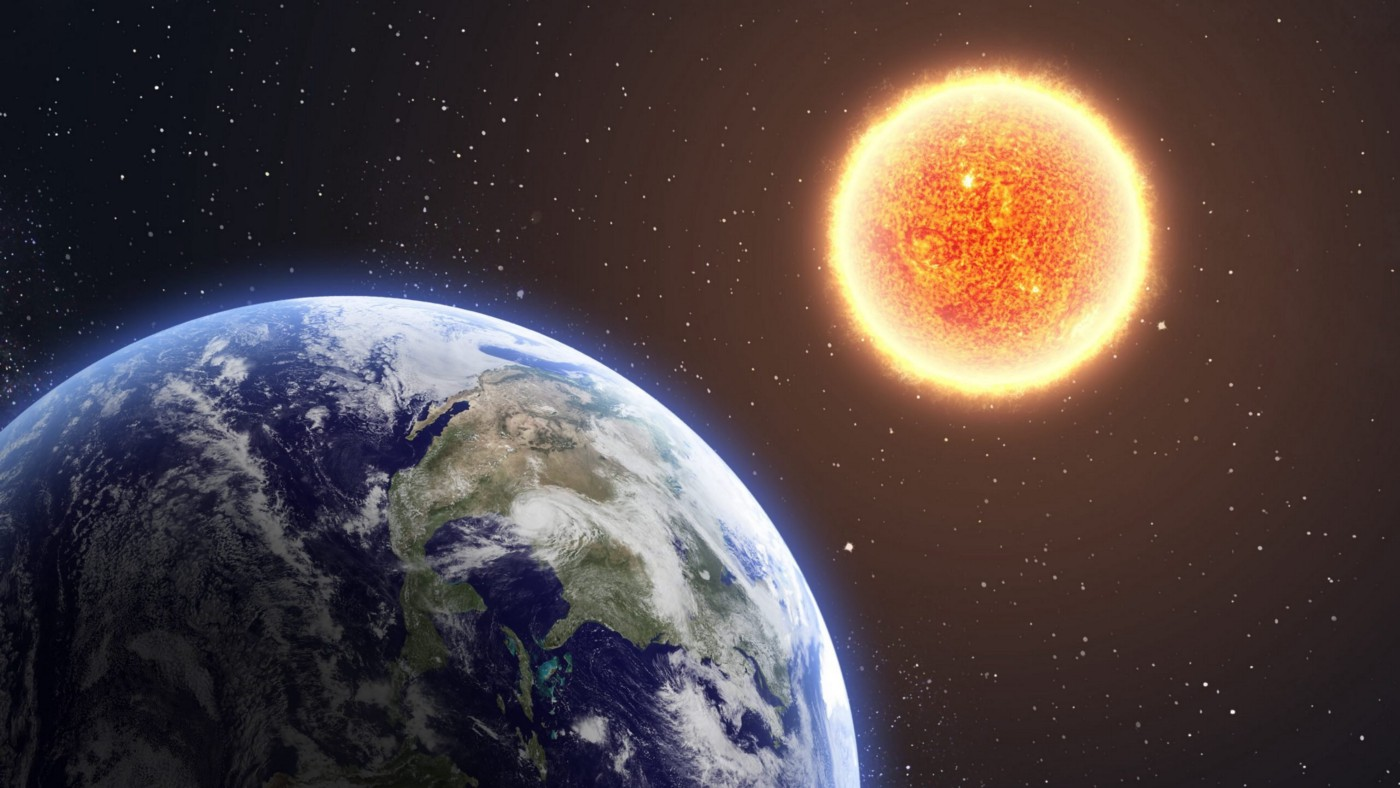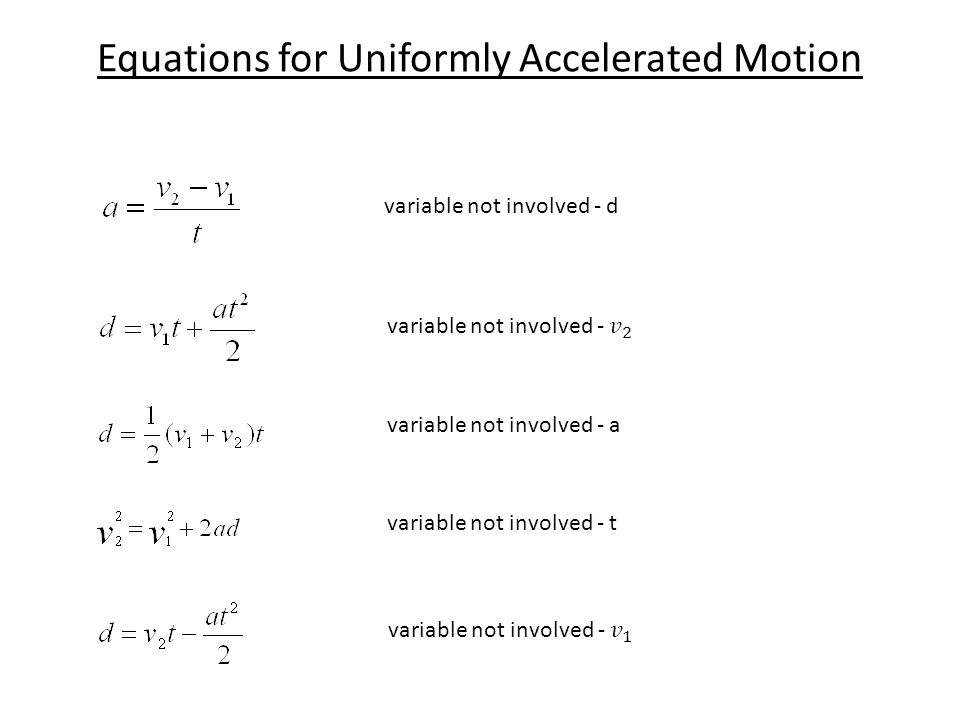# Displacement During Uniform Acceleration Study Guide

INTRODUCTION

Have you ever thought why, despite the earth’s rotation around the sun in a spherical orbit, you never feel any movement when on the planet? There is, after all, a logic to it. The uniform acceleration that occurs enables the movement that you cannot feel. Let’s learn about this in detail.Source

## DISPLACEMENT

• To understand an object’s motion, we need to understand its position and the change in its position.
• Definition of displacement is the change in the position of an object.
• The position of an object changes when it shifts relative to a reference frame.
• Displacement is the term for this shift in position.
• Examples of displacement can be – when a soccer ball moves to the right relative to a player or when a person moves toward the back of a car.
• Also, a displacement per unit of time is the velocity of the object in motion.

## ACCELERATION

• Any process in which the velocity varies is referred to as acceleration.
• Because velocity is both a speed and a direction, you can only accelerate in one of two ways: alter your speed or modify your direction—or both.
• Acceleration is a vector quantity that is described as the rate of change in velocity.
• The acceleration can be positive, negative, or zero depending upon the velocity and its direction.

## UNIFORM ACCELERATIONSource

• We’re all aware of the concept of uniform motion: a body is said to be in uniform motion if it travels the same distance in the same amount of time.
• The meaning of uniform acceleration can be that when an object moves in a single direction and its net velocity changes with time remain constant.
• A cycle running down a hill and a child tumbling down the slide are some of the common examples of uniform acceleration.

The concept of displacement during uniform acceleration has three equations that relate the displacement with the other quantities in the uniform accelerated motion.

s= ut+1/2 a t2

v= u+at

And, v2= u2+2as

Where,

v – The final Velocity

u – The initial Velocity

a – Acceleration

s – The displacement

t – The time interval

# SUMMARY

• Displacement is the change in position of an object
• The rate at which your velocity changes are called acceleration, and it is a vector quantity.
• A body is in uniform acceleration when it moves in a single direction, and its velocity does not change with time.

## FAQs

1. How does acceleration affect displacement?

Velocity is the rate at which displacement changes; it’s just how quick and in which direction you’re going. The rate at which your velocity changes is called acceleration.

2. Does acceleration use displacement?

Yes, Acceleration is directly related to velocity, which is the rate of change in displacement

3.What happens during uniform acceleration?

A body is in uniform acceleration when it moves in a single direction, and its velocity does not change with time.

We hope you enjoyed studying this lesson and learned something cool about Displacement during Uniform Acceleration! Join our Discord community to get any questions you may have answered and to engage with other students just like you! We promise, it makes studying much more fun!😎

## REFERENCE

1. Displacement During Uniform Acceleration: https://flexbooks.ck12.org/cbook/ck-12-physics-flexbook-2.0/section/2.6/primary/lesson/displacement-during-constant-acceleration-phys/. Accessed 8th April 2022.
2. Finding Velocity and Displacement From Acceleration: https://courses.lumenlearning.com/suny-osuniversityphysics/chapter/3-6-finding-velocity-and-displacement-from-acceleration/Accessed 8th April 2022.
]]>